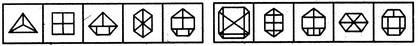# Non Verbal Reasoning - Series - Discussion

Discussion Forum : Series - Section 1 (Q.No. 12)
Directions to Solve

Each of the following questions consists of five figures marked A, B, C, D and E called the Problem Figures followed by five other figures marked 1, 2, 3, 4 and 5 called the Answer Figures. Select a figure from amongst the Answer Figures which will continue the same series as established by the five Problem Figures.

12.

Select a figure from amongst the Answer Figures which will continue the same series as established by the five Problem Figures.(A)     (B)     (C)     (D)     (E)       (1)     (2)     (3)     (4)     (5)
1
2
3
4
5
Explanation:
The number of parts increases by one along with the number of sides in the figure.
Discussion:
28 comments Page 1 of 3.

Jankar said:   1 month ago
Explanation:

The number of edges increases by "1 " in each figure.

1. Figure "A" has 3 edges & 3 divisions.
2. Figure "B" has 4 edges & 4 divisions.
3. Figure "C" has 5 edges & 5 divisions.
4. Figure "D" has 6 edges & 6 divisions.
5. Figure "E" has 7 edges & 7 divisions.

Therefore the next figure should have"8" edges & 8 division.
-The figure "5" in the answer column has 8 edges & division.
Hence "E" is the correct answer.

Happil said:   3 years ago
Ans: E. (5)

Explanation:
The number of edges increases by "1 " in each figure.

1. Figure "A" has 3 edges.
2. Figure "B" has 4 edges.
3. Figure "C" has 5 edges.
4. Figure "D" has 6 edges.
5. Figure "E" has 7 edges.

Therefore the next figure should have"8" edges
-The figure "5" in the answer column has 8 edges.
Hence "E" is the correct answer.
(1)

Sai said:   3 years ago
A and E have 8 sides. How can you declare only E? Please explain.
(1)

Shru said:   4 years ago
Thanks @Ajay.

By combining Fig -A and B we get Fig C and also the no of sides are also included.

Samarth said:   4 years ago
Figure B also has 8 sides. What should we do now?

Kushal pagdhare said:   5 years ago
1st option also have 8 sides.

Bank said:   6 years ago
You are correct. Thanks @Ajay.

Shubham said:   7 years ago
Option A & E have same number of sides.

Rshikesh said:   7 years ago
I think B also be an answer.